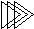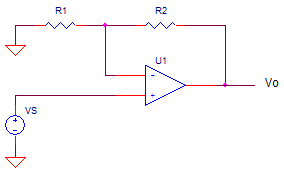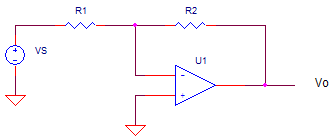Electronic Design with Excel
 Topics | VBA Basics | Back to eCircuit Center

OP AMP GAIN AND BANDWIDTH

OP AMP GAIN

Although the VBA code for this topic is simple, I found the insight into gain and bandwidth useful. Assuming you've been around the electronics neighborhood, you've seen the two main op amp players: the non-inverting amplifierand the inverting amplifierThe gain (K = vo/vs) for each of these amplifiers is given by

NON-INVERTING:   K_non = R1 / R2 +1
INVERTING:           K_inv  = -R2 / R1

Let's create some VBA functions to perform these calculations . (Also see VBA Basics). To see the VBA code, hit ALT-F11 and double click on the Modules > Module1 in the VBA Project window. This opens the code window for this module.

 Function K_op_non(R1, R2)    ' Op amp closed loop gain - non-inverting amplifier    K_op_non = (R2 + R1) / R1 End Function Function K_op_inv(R1, R2)    ' Op amp closed loop gain - inverting amplifier    K_op_inv = -R2 / R1 End Functionn

GAIN

For the non-inverting and inverting  amplifier, what is the gains given R1 and R2? First, we calculate the gain for both configurations. For example, the non-inverting gain is calculated in the Knon column by function =K_op_non(B18,C18). Here are the first several gain calculations for each configuration.

 Non-Inverting Amplifier R1 R2 K non fbw 1.00E+09 1,000 1.0 999,999 1,000 1,000 2.0 500,000 1,000 9,000 10.0 100,000

 Inverting Amplifier R1 R2 K inv K non fbw 1,000 1,000 -1.0 2.0 500,000 1,000 2,000 -2.0 3.0 333,333 1,000 10,000 -10.0 11.0 90,909

For comparison, I chose R1 and R2 to give the gains 1,2,10,100,1000 for the non-inverting and -1,-2,-10,-100,-1000 for the inverting. Note, for a non-inverting gain of 1, you need R1 open or R1 = infinity ohms. Because Excel has no such value, I entered 1 G ohm as a practical alternative.

Also note, the resistor ratios are different for same magnitude of gain. As an example:
for K_non=2, you need R1=R2=1k. However, for K_inv = -2, you need R1 = 1k and R2 = 2k.

BANDWIDTH

Another big question is this - what is the bandwidth of our amplifiers? For the non-inverting amplifier, the bandwidth is approximated by

f_bw = fu / Knon                 Non-Inverting Bandwidth

where fu is the Unity Gain Bandwidth or more commonly called the Gain Bandwidth Product (GBP) in data sheets. The above equation says that your device's bandwidth (fu or GBP) is essentially knocked down by your gain!  Oh, the pain! The more gain you need, the less bandwidth you get!

What do you expect happens to the bandwidth of the inverting amplifier? It can be approximated by

f_bw = fu / Knon                 Inverting Bandwidth

But, wait! You say there must be some typo - shouldn't it read fbw = fu / Kinv ? No, unfortunately. For the inverting amp, fu is divided by the equivalent non-inverting gain of the amplifier! There's an extra column in the inverting amp section to calculate the equivalent Knon.

Overall, this is bad news for the inverting amp. Why? For a gain of Kinv = -1 (given R1=R2=1k), it's non-inverting gain is Knon=R2/R1+1 = 2. Even though it has gain magnitude of 1, its bandwidth gets knocked down by a factor of 2.

In general, if you need more bandwidth, choose an op amp with a higher fu (GBP). Try this by increasing fu by a factor of 2 to 10 or so, then see the effect on all of the bandwidth calculations.

The remainder of the Excel file shows that for the same magnitude of gain, the non-inverting amp has a higher bandwidth. So for all other things being equal, choose the non-inverting amp. However, you'll notice for higher gains, the advantage becomes less and less as R2/R1 ~ R2/R1+1 for R2/R1 >> 1.

The best part of Excel / VBA is that it lets you play and experiment. Jump right in and start messing with it or write a new function altogether. Just copy the existing code to a space just below the original. Or, open a new sheet and play with the existing functions. Here are a few ideas you can try:

1. Use the functions to calculate the effect of resistor tolerances on gain. For example, enter "K_op_non(B18*1.01, C18)" to see the impact of 1% resistor error.

2. Use the functions to calculate an output voltage based on the input and gain. Add a column for vs and vo, then use the Knon column to calc vo based on vs.